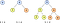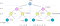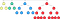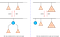# A Purely Functional Random Access List in Scala

In this article, we present a Scala implementation of a purely functional random access list; a functional data structure which was first presented by Chris Okasaki in this paper.

The random access list data structure supports array lookup and update operations in O(log n) time, and simultaneously provide O(1) time cons, tail and head list operations.

Lists are central to functional programming. They are convenient and well suited to implement recursive algorithms. However, for applications which need random access to data, they become inefficient, because accessing the iᵗʰ element in a list, requires O(i) time. Arrays, on the other hand, are very fast when it comes to randomly looking-up elements, but they can prove very awkward in a functional programming setting. Arrays are mutable data structures which destruct their data once updated. Also, their efficiency is tied to the assumption that they are used in a single-threaded environment. To that end, random access lists are the best of both worlds. They are immutable data structures that can be used safely in multithreaded environments without sacrificing the random access efficiency. Next, we’ll see how that can be achieved.

# Complete Binary Trees

A complete binary tree is a binary tree which has a root node and two totally balanced subtrees, the left subtree and the right subtree. Thus, the number of nodes in a complete binary tree is a skew binary number of the form 2ᵏ -1, where k is a positive integer (see figure 1).Figure 1. Complete binary trees for k = 1, 2, and 3.

As the complete binary tree will be our basic building block in implementing the random access list, we’ll start by implementing the complete binary tree as an algebraic data type.

First we must understand how values are stored in a complete binary tree. For a list of values of size n, (x₀, x₁, …, xn-₁), where n is a skew binary positive integer, x₀ is stored at the tree root, x₁… xn/₂ are stored at the left subtree, and x(n/₂)+₁… xn-₁ are stored at the right subtree (see figure 2).Figure 2. Storing values in a complete binary tree.

Other arrangements are possible, but this particular arrangement will prove advantageous in our implementations of basic array and list operations.

A complete binary tree may be represented in code as¹:

The `CompleteBinaryTree` polymorphic type is represented as a `sealed trait`(listing 1). Thus, all data constructors of `CompleteBinaryTree` are required to be declared here; namely `Node` and `Leaf`. A tree of type `Leaf` is a singleton tree. A tree of type`Node` contains two subtrees: a lift subtree and a right subtree. Since, in this article, we are only concerned about complete binary trees, which are totally balanced, the two subtrees of a `Node` must be either `Node`s or `Leaf`s.

Now, we consider the implementations of the two basic array operations: `lookup()` and `update()`. The `lookup()` function uses pattern matching to produce the output value depending on the type of the `CompleteBinaryTree` and the `index` of the value being searched for. If the `CompleteBinaryTree` is a `Leaf`, the value contained in the`Leaf` is returned as its the only value there is. However, if the `index` supplied to `lookup()` is not `0`, an exception is thrown, because a `Leaf` is a singleton tree. If the `CompleteBinaryTree` is a `Node`, the value at the root of the tree is returned if the `index` supplied to `lookup()` is `0`. If `index` is not `0`, and `index``size / 2` , where `size` is the size of the tree, then the required value must be in the left subtree (see figure 2). In this case `lookup()` calls itself recursively supplying the left subtree as the tree together with updated values for `index` and `size`. Finally, if `index` > `size / 2`, `lookup()` , again, calls itself, recursively. Only this time, the supplied values to the call is the right subtree and updated values for `index` and `size`.

The `update()` function works in similar way to the `lookup()` function, but it returns a new `CompleteBinaryTree` updated at the `index` supplied in the call with the new value specified by in same call. If the tree being updated is a `Leaf`, a new `Leaf` is returned with its only value updated to match the new value supplied by the caller. However, that is only possible, if `index` is `0`, otherwise an exception is thrown as before. If the tree is a `Node`, `index` is checked to determine which subtree is going to be updated. The `update()` function returns a new `Node`, which consists of the root of the old `Node`, one of the two old subtrees depending on the values of `index` and `size`, and an updated subtree generated by a recursive call to `update()`. Note that `update()` always return a new data structure and doesn’t mutate the old data structure. This immutable approach to updating data structures is an essential pillar of pure functional algorithm design.

It is worth noting that because the height of a complete binary tree is logarithmic in its size (see figure 2), the two array operations run in O(log n) time.

The companion object to `CompleteBinaryTree` declares a convenient function, `apply()`, which is useful in constructing a `CompleteBinaryTree` from an ordinary `List`. Function `apply()` checks the size of the input list, to determine if the list has one or more elements. If there is only one element in the list, a `Leaf` is generated to wrap the only value in the list. If there are more than one element in the list, the head of the list is used to populate the root of a `Node` . The tail of the list is, then, divided into two equal lists which are used to construct the left and right subtrees of the `Node` through two recursive calls to `inner()`. Note that function `inner()` is used by `apply()` to do the heavy lifting. The convenient Scala syntax `CompleteBinaryTree(List(....))` is used when calling `apply()`, which will throw an exception if the length of the supplied list is not a skew binary number. The length of the list is calculated once by `apply()` and then supplied to `inner()` to avoid the cost of calculating the list length several times.

It might be tempting to think that a `CompleteBinaryTree` is enough to construct our target data structure, the random access list, but the limitation on the size of the input list is a serious one. We need to have a way to start with an ordinary list of arbitrary size and end up with a random access list. We’ll see how, next.

# A Full-Fledged Random Access List

To represent lists of arbitrary sizes, one can utilize a list of complete binary trees instead of just one tree. We know this is possible, because an empty list can be represented by an empty list of complete binary trees and any other list of arbitrary size > 0 can be represented by size number of singleton trees. However, we are going to use one specific decomposition of size called the greedy skew-binary decomposition (GSBD).

A skew-binary decomposition of a natural number is said to be greedy if the largest term in the decomposition is as large as possible and the rest of the decomposition is also greedy. For example, the GSBD of 28 is [3, 3, 7, 15], as shown in figure 3.Figure 3. Greedy skew-binary decomposition of 28.

The above recursive definition of GSBD can be used to devise an algorithm to generate g(n), the GSBD of the natural number n. The algorithm is straightforward as shown by the below Scala code:

`package greedySkewBinaryobject Gen {  def g(n: Int): List[Int] = {    def loop(n: Int, acc: List[Int]): List[Int] = {      import Math._      if (n == 0) acc else {        val term = pow(2, floor(log(n + 1) / log(2))).toInt - 1        loop(n - term, term::acc)      }    }    loop(n, List())  }}`

Function `g()` accepts an `Int`, `n`, for which it outputs the GSBD as a `List[Int]`. Internally, `g()` uses a function `loop()` to do the actual work. Function `loop()` uses an accumulator, `acc`, to memorize the decomposition `term`s between its successive calls to itself. When `n == 0`, `acc` is returned, otherwise, `loop()` calculates the next term of the decomposition and calls itself supplying `n — term` as the new `Int` to be decomposed, together with an `acc` that has been updated with the newly calculated `term`. All `g()` has to do, is to call `loop()` supplying `n` as the `Int` to be decomposed an and empty `List` as the initial value of the accumulator.

Despite the simplicity of the above algorithm, it can be used to deduce some important properties of GSBDs, in general:

1. The algorithm is deterministic. In other words, it will always produce a decomposition no matter the value of n as long as n ≥ 0. Thus, every natural number has a greedy skew-binary decomposition.
2. Let us assume that there exist two different GSBDs of a natural number n, g₁(n) and g₂(n). By definition, the largest term in g₁(n), T₁, and the largest term in g₂(n), T₂, must be the same. The remaining of the two decompositions, g₁(n-T₁) and g₂(n-T₂), are also greedy, by definition. Hence, the second largest term in g₁(n) and the second largest term in g₂(n) must be also the same, and so on. We conclude that g₁(n) and g₂(n) must be the same. Thus, every natural number has a unique greedy skew-binary decomposition.
3. Let us assume that two successive terms tᵢ, and tᵢ+₁ of the GSBD of n, g(n), are equal. In the algorithm that we used before to generate g(n), this means that tᵢ+₂ is calculated by supplying (tᵢ+₁)-tᵢ to the next iteration of the algorithm as the number to be decomposed. But, (tᵢ+₁)-tᵢ = 0; hence, the algorithm terminates. Thus, all terms of a greedy skew-binary decomposition are unique except for the two smallest terms (the first two terms in the ascending ordering of the decomposition terms), which may be equal.
4. We have shown before that we can do lookup and update operations on a complete binary tree in O(log n) time. But how about if we want to lookup or update a value in a list of complete binary trees? Well, it turns out that if the list terms are the GSBD of the list size, we are still able to do lookup and update operations in O(log n) time. To show that this is possible, we must find the upper limit on the number of terms in the GSBD of n, g(n). We know that g(n) terms are all skew-binary numbers ≤ n. We also know from property 3 above, that they must be all unique except for the smallest two of them which may be repeated. Assuming that g(n) contains all the skew-binary numbers which are > 0 and ≤ n with the smallest term repeated, g(n) will have k+1 terms where 2ᵏ-1 is the largest skew binary number ≤ n. Because we know that k is an integer, k + 1=log₂(n+1) + 1. Note that for log₂(n+1) to be an integer, n must be a skew-binary number, in which case g(n) will consist of only one term, namely, 2ᵏ-1. Obviously, this case is not useful in our search for the upper limit on the number of terms in g(n). We can safely assume that log₂(n+1) is not an integer. Thus, the upper limit, we are looking for is k + 1=log₂(n+1) + 1 = log₂(n+1)⌉. Thus, the maximum number of trees in the list, k+1, is logarithmic in the total number of elements n, which means that locating the right tree for lookup and update operations can be done in O(log n) time.
5. Finally, we consider the relation between g(n) and g(n+1). This is important because it gives us a way to implement two important list operations, cons() and tail(). Let g(n) = [t₀, t₁, …, T₁, T₀] and g(n + 1) = [t₀`, t₁`,…, T₁`, T₀`]. If T₀≠T₀`, then n+1 must be a skew-binary number and hence g(n) = [n/2, n/2] and g(n+1) = [n + 1]. If T₀=T₀`, then g(n-T₀) = [t₀, t₁, …, T₁] and g(n+1-T₀)=[t₀`, t₁`,…, T₁`]. If T₁≠T₁`, then n+1-T₀ must be a skew-binary number and hence, g(n-T₀)=[(n-T₀)/2, (n-T₀)/2] and g(n+1-T₀)=[(n-T₀)+1], and so on. We conclude that, if t₀ = t₁, then g(n+1) = [[1,t₀, t₁], t, ..]; and if t₀ ≠ t₁, then g(n+1)=[1,t₀, t₁,…].

We can take advantage of property 5 to implement efficient cons() and tail() operation. In cons(), we are adding an element to the front of the list. If the GSBD of the original list’s size is g(n) then the GSBD of the resulting list’s size is g(n+1). The opposite is true in the case of tail(). If the GSBD of the list’s size is g(n+1), then the GSBD of it tail’s size is g(n) (see figure 4).Figure 4. How cons() and tail() operations work on a random access list.

Now, it is time to show the code for the `RandomAccessList` (listing 2).

As seen in listing 2, `RandomAccessList` is just a `type` alias for `List[(CompleteBinaryTree, Int)]`. The `Int` type parameter is the size of the `CompleteBinaryTree`. Function `fromList()` transforms an ordinary `List` into a `List` of `List`s. Each sublist is a `CompleteBinaryTree` of size equal to the corresponding term in the GSBD of the input `List`’s size. The previously mentioned algorithm which generates GSBDs, is used by `toList()` to generate the `CompleteBinaryTree`s (the sublists of the output `List`).

Function `lookup()` uses the supplied `index` to check if the element being searched for is in the first subtree. If so, it calls `CompleteBinaryTree`’s `lookup()` function to return the element. If not, it calls itself supplying the tail of the input `List` together with an updated value of `index`. Function `update()` works in a similar way to `lookup()`, but it calls `CompleteBinaryTree`’s `update()` function, instead of its `lookup()`. Both `lookup() `and `update()` run in O(log n) time. O(log n) to locate the right subtree and O(log n) to locate the right element within the subtree.

If the first two elements of the input `List` are equal, `cons()` turns them into the left and right trees of a newly created `Node`, respectively. At the root of the `Node`, `cons() `stores the element supplied by the caller, `e`.The newly created `Node` is the first element in the resulting `RandomAccessList`. If the input `List` has more elements beyond the second element, they will represent the tail of the `RandomAccessList`; otherwise the tail will be an empty `List` (`Nil`). If the first two elements of the input `List` are not equal, or if the input `List`’s size is < 2, the whole input list will represent the tail of the resulting `RandomAccessList`, while its head will be a `Leaf` containing `e`. Note that `cons()` requires O(1) running time.

Function `head()` also requires O(1) running time and will always return the value contained by the first element in the input `List` (except when the input `List` is `Nil`, in which case an exception is thrown).

Function `tail()` checks if the head of the input `List` is a `Leaf`. If so, it just returns the the input `List`’s tail. Otherwise, if the head of the input `List` is a `Node`, a `RandomAccessList` is returned with the left and right trees of `Node` representing the first and the second element of the resulting `RandomAccessList`, respectively; and the tail of the input `List` representing the remaining elements of the `RandomAccessList`. Clearly, `tail()` requires O(1) running time.

We conclude that list operations on a `RandomAccessList` require O(1) time while array operations require O(log n) time. It gets even better when we note that locating the iᵗʰ element in a `CompleteBinaryTree` requires O(log i) time. Also, if we assume that a `RandomAccessList` contains one `CompleteBinaryTree `of every size < n, then the iᵗʰ element of the list will be in the log(i)ᵗʰ tree, approximately. Which means that locating the right tree requires O(log i) time, too. In fact, this approximation remains good even if we have some missing trees but not if we have large gaps. While it can be shown that the probability of having large gaps is very low for randomly chosen n, we feel it is intuitive. Thus, array operations on `RandomAccessList` require O(log i) time in the expected case and O(log n) time in the worst case.